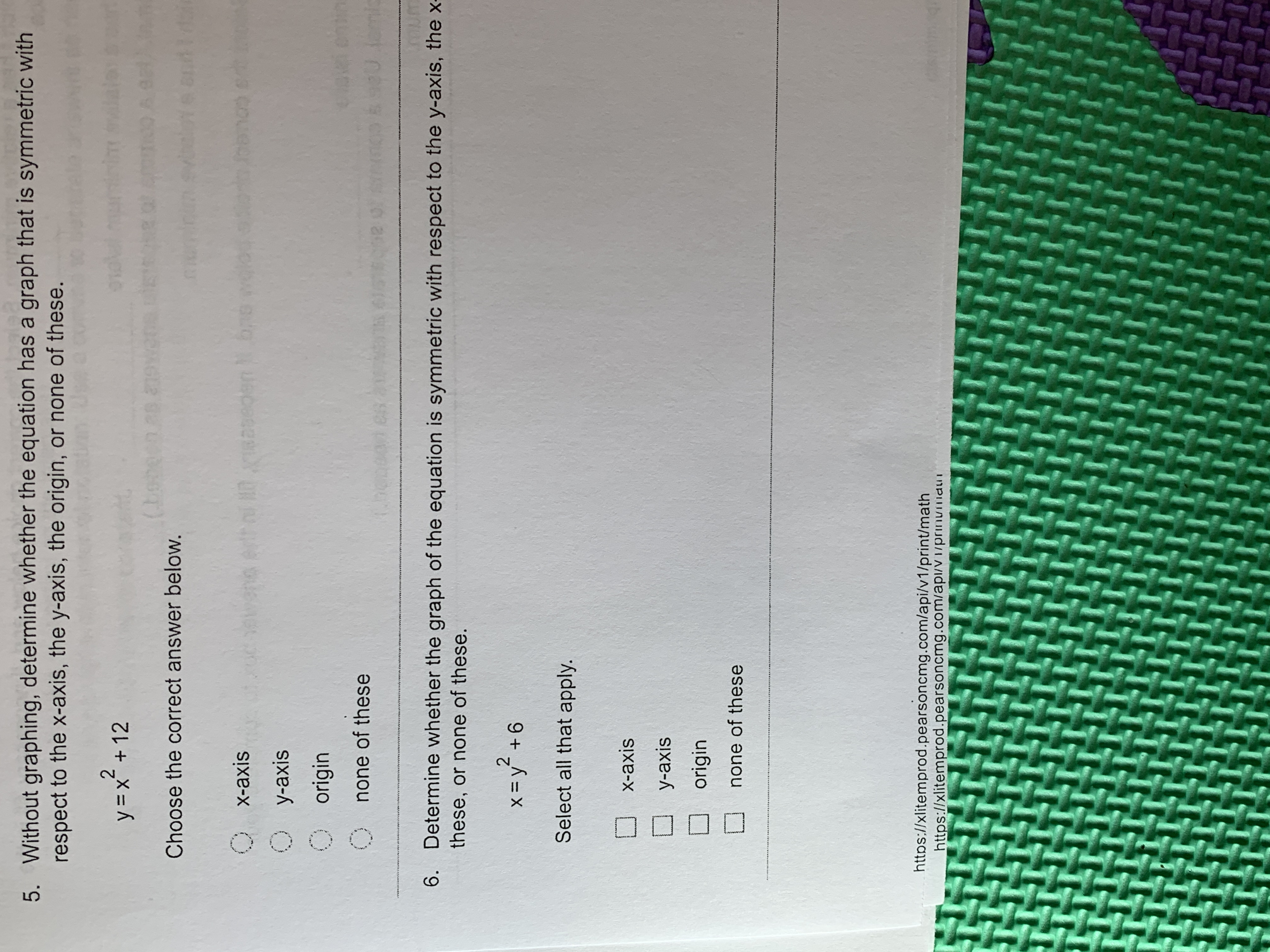# 5. Without graphing, determine whether the equation has a graph that is symmetric withrespect to the x-axis, the y-axis, the origin, or none of these.2y x125.(bobern esChoose the correct answer below.0 X-axisMO J8se0en1b0 y-axisOoriginn(Onone of these6. Determine whether the graph of the equation is symmetric with respect to the y-axis, the xthese, or none of these.2X=y +6Select all that apply.X-axisУ-аxisoriginnone of thesehttps://xlitemprod.pearsoncmg.com/api/v1/print/mathhttps://xlitemprod.pearsoncmg.com/api/vI/prinumiaur

Question

Can someone help me solve this problem? Number fivehelp_outlineImage Transcriptionclose5. Without graphing, determine whether the equation has a graph that is symmetric with respect to the x-axis, the y-axis, the origin, or none of these. 2 y x12 5. (bobern es Choose the correct answer below. 0 X-axis MO J8se0en1b 0 y-axis Oorigin n (O none of these 6. Determine whether the graph of the equation is symmetric with respect to the y-axis, the x these, or none of these. 2 X=y +6 Select all that apply. X-axis У-аxis origin none of these https://xlitemprod.pearsoncmg.com/api/v1/print/math https://xlitemprod.pearsoncmg.com/api/vI/prinumiaur fullscreen
check_circleExpert Solution
Step 1

Given function is

Step 2

For symmetry about X- axis, we replace y by -y and if the equation is same to the original equation then function is symmetric about X- axis

Step 3

For symmetry about Y- axis, we replace x by -x and if the equation is same...

### Want to see the full answer?

See Solution

#### Want to see this answer and more?

Solutions are written by subject experts who are available 24/7. Questions are typically answered within 1 hour*

See Solution
*Response times may vary by subject and question
Tagged in

### Other